Next: Spontaneous Emission Up: Time-Dependent Perturbation Theory Previous: Electromagnetic Radiation

# Electric Dipole Approximation

In general, the wavelength of the type of electromagnetic radiation which induces, or is emitted during, transitions between different atomic energy levels is much larger than the typical size of an atom. Thus,(1109)

can be approximated by its first term, unity. This approach is known as the electric dipole approximation. It follows that(1110)

Now, it is readily demonstrated that(1111)

so(1112)

Thus, our previous expressions for the transition rates for radiation induced absorption and stimulated emission reduce to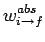(1113)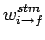(1114)

respectively. Here,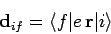(1115)

is the effective electric dipole moment of the atom when making a transition from state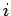to state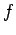.

Equations (1113) and (1114) give the transition rates for absorption and stimulated emission, respectively, induced by a linearly polarized plane-wave. Actually, we are more interested in the transition rates induced by unpolarized isotropic radiation. To obtain these we must average Eqs. (1113) and (1114) over all possible polarizations and propagation directions of the wave. To facilitate this process, we can define a set of Cartesian coordinates such that the wavevector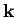, which specifies the direction of wave propagation, points along the-axis, and the vector, which specifies the direction of the atomic dipole moment, lies in the-plane. It follows that the vector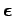, which specifies the direction of wave polarization, must lie in the-plane, since it has to be orthogonal to. Thus, we can write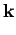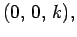(1116)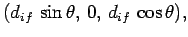(1117)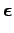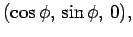(1118)

which implies that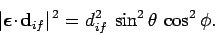(1119)

We must now average the above quantity over all possible values ofand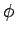. Thus,(1120)

where, and the integral is taken over all solid angle. It is easily demonstrated that(1121)

Here,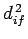stands for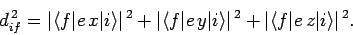(1122)

Hence, the transition rates for absorption and stimulated emission induced by unpolarized isotropic radiation are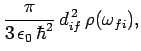(1123)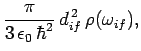(1124)

respectively.Next: Spontaneous Emission Up: Time-Dependent Perturbation Theory Previous: Electromagnetic Radiation
Richard Fitzpatrick 2010-07-20